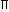# Aptitude - Volume and Surface Area - Discussion

### Discussion :: Volume and Surface Area - General Questions (Q.No.8)

8.

The slant height of a right circular cone is 10 m and its height is 8 m. Find the area of its curved surface.

 [A]. 30m2 [B]. 40m2 [C]. 60m2 [D]. 80m2

Explanation:

l = 10 m,

h = 8 m.

So, r = l2 - h2 = (10)2 - 82 = 6 m.Curved surface area =rl = (x 6 x 10) m2 = 60m2.

 Viveka Shahi said: (Sep 5, 2014) But R is not radius. It is diameter of the right circular cone. So answer should be [A].

 Sunil said: (Nov 19, 2015) Curved surface area of cone = rl. r stands for radius. l stands for slant height. r = ? height = 8 m. l = 10 m. First we should find to r (radius). Then we can find curved surface area of cone. r = l - r. r = 10-8 = 6 m. Put value of r in given formula. rl = 6 m*10 m = 60 m^2. (C) 60 m^2 correct option.

 Subhasis said: (Feb 24, 2016) I think r should be 6/2 = 3. What is the right answer?

 Islam said: (Mar 7, 2016) C is correct. l = 10 m, h = 8 m. So, r = l2 - h2 = (10)2 - 82 = 6 m. Curved surface area = rl = ( x 6 x 10) m2 = 60 m2.

 Krutarth Vaidya said: (Jun 22, 2016) C is also correct. L = 10, H = 8, So, r = l2 - h2 = (10)2 - 82 = 6 m. Area = 60 m^2.

 Gowtham said: (Jan 15, 2017) @Sunil. How 10-8 = 6? It should be 2.

 Justin said: (Feb 25, 2017) Answer is A, why you are taking as a full height of 10? We need a radius only, I think; (22/7) x 6 x 5, (22/7) x 30 => is a correct Answer.

 Mustafa said: (Mar 30, 2017) Yeah, 10-8 should be 2 not 6.

 Nikita said: (Apr 1, 2017) L = 10 m. H = 8 m. R = (10 * 10) - (8 * 8). R = 100 - 64 = 36. Square root of 36 is 6. = 6 * 10 = 60m.

 Ngawang Chojey said: (May 15, 2018) @Nikita is right. You can use the formula of a right triangle to derive the length of base i.e. equal to radius: h^2=a^2+b^2. Where, h=slant height, a=hight of a right triangle and b=base of a right triangle.